# excel 这也许是史上最好最全的VLOOKUP函数教程

2019/07/09 14:48

1、根据番号精确查找俗称。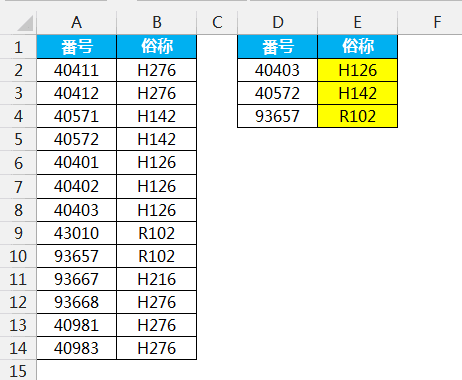=VLOOKUP(D2,A:B,2,0)

VLOOKUP函数语法：

=VLOOKUP(查找值,查找区域,返回查找区域第N列,查找模式)

VLOOKUP函数示意图。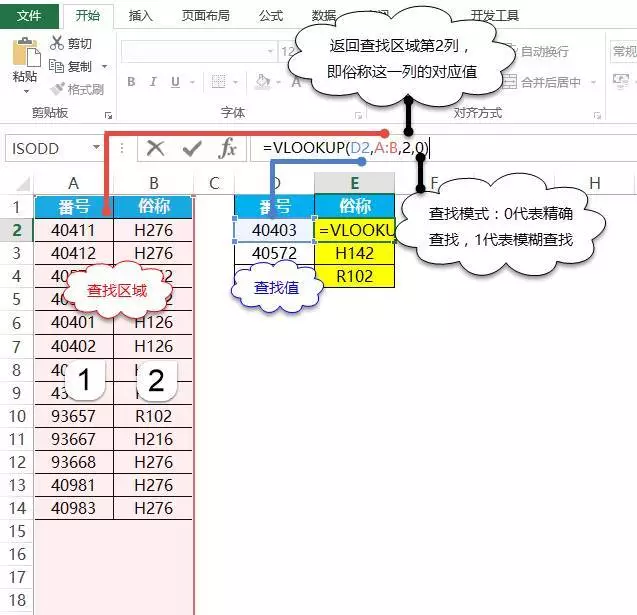2、屏蔽错误值错误值查找。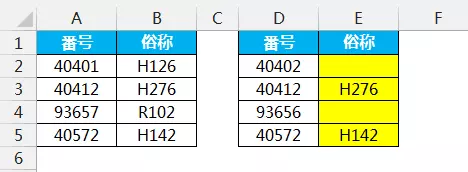=VLOOKUP(D2,A:B,2,0)

VLOOKUP函数如果查找不到对应值会显示错误值#N/A，这个看起来很不美观。这时可以在外面加个容错函数IFERROR，如果是2013版本那就更好，可以用IFNA函数，这个是专门处理#N/A这种错误值。

=IFERROR(VLOOKUP(D2,A:B,2,0),"")=IFNA(VLOOKUP(D2,A:B,2,0),"")

=IFERROR(表达式,错误值要显示的结果)

3、按顺序返回多列对应值。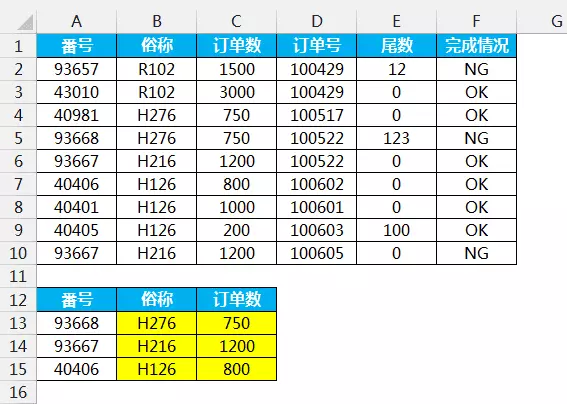=VLOOKUP(\$A13,\$A\$1:\$F\$10,2,0)

=VLOOKUP(\$A13,\$A\$1:\$F\$10,3,0)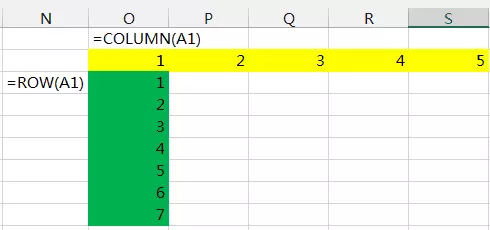=VLOOKUP(\$A13,\$A\$1:\$F\$10,COLUMN(B1),0)

4、按不同顺序返回对应值。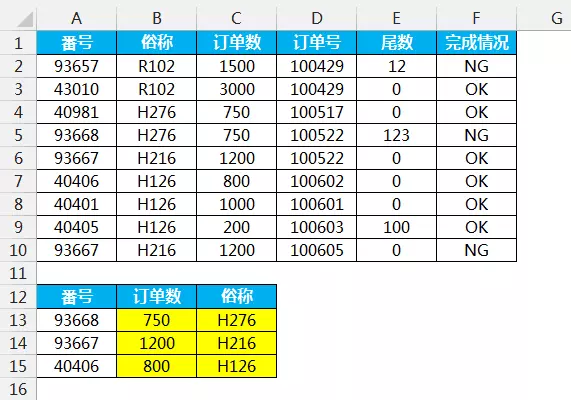NO！每当你觉得操作繁琐时，就要停下来思考，也许Excel本身存在这个功能，只是自己一时想不到或者不知道而已。列号不管千变万化，在数据源的位置始终不变，利用这个特点可以去搜索一下看看有什么函数可以解决。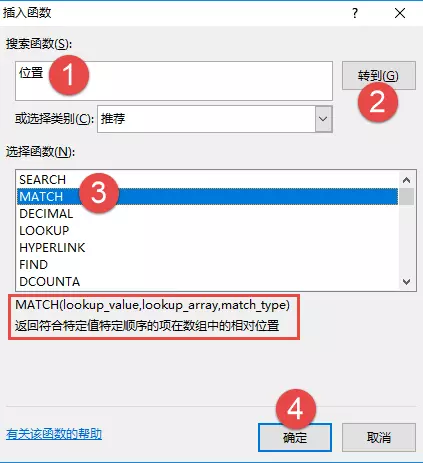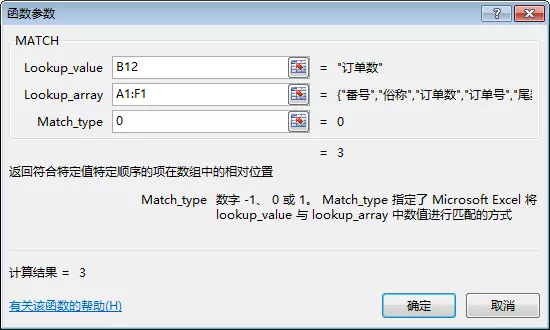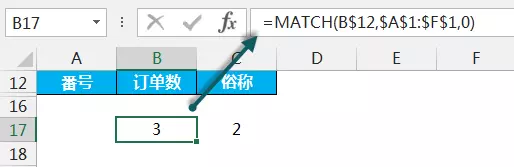=VLOOKUP(\$A13,\$A\$1:\$F\$10,MATCH(B\$12,\$A\$1:\$F\$1,0),0)

5、根据番号逆序俗称。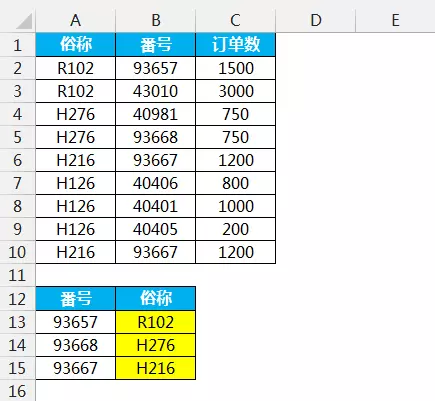IF函数其实只有一个条件来判断是否符合条件，返回FALSE和TRUE两种结果。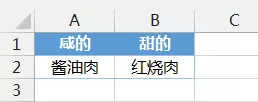=IF(A1="咸的",A2,B2)

A1="咸的"这个条件也可以直接换成TRUE或者FALSE。

=IF(TRUE,A2,B2)

=IF(FALSE,A2,B2)

=IF(1,A2,B2)=IF(0,A2,B2)

IF函数不止可以返回1个单元格的值，也可以返回多个单元格的值。

=IF({1,0},A2,B2)=IF({0,1},A2,B2)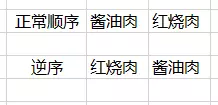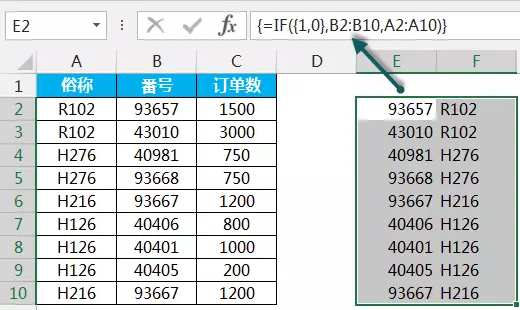=IF({1,0},B2:B10,A2:A10)

=VLOOKUP(A13,新区域,2,0)

=VLOOKUP(A13,IF({1,0},\$B\$2:\$B\$10,\$A\$2:\$A\$10),2,0)

6、根据俗称跟订单号两个条件查询完成情况。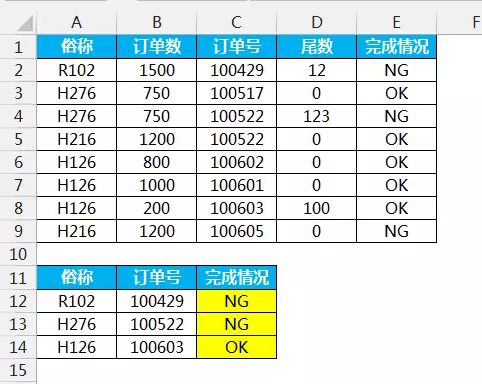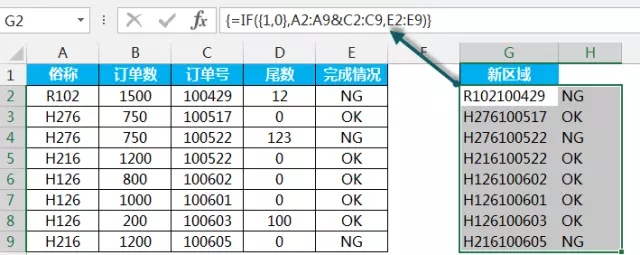=IF({1,0},A2:A9&C2:C9,E2:E9)

=VLOOKUP(A12&B12,新区域,2,0)

=VLOOKUP(A12&B12,IF({1,0},\$A\$2:\$A\$9&\$C\$2:\$C\$9,\$E\$2:\$E\$9),2,0)

7、根据俗称的第一个字符查找番号。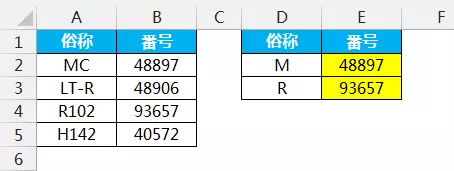=VLOOKUP(D2&"*",A:B,2,0)

8、根据区域判断成绩的等级。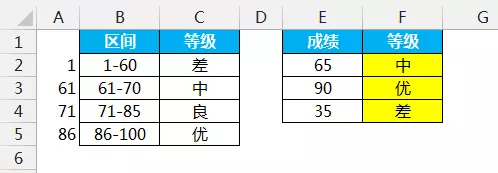=VLOOKUP(E2,A:C,3)

=VLOOKUP(E2,IF({1,0},--LEFT(B\$2:B\$5,FIND("-",B\$2:B\$5)-1),C\$2:C\$5),2)

=VLOOKUP(E2,IF({1,0},IMREAL(B\$2:B\$5&"i"),C\$2:C\$5),2)

IMREAL函数是计算复数的实部系数的函数，作用就是提取区间的下限。

0
1 收藏

### 作者的其它热门文章0 评论
1 收藏
0